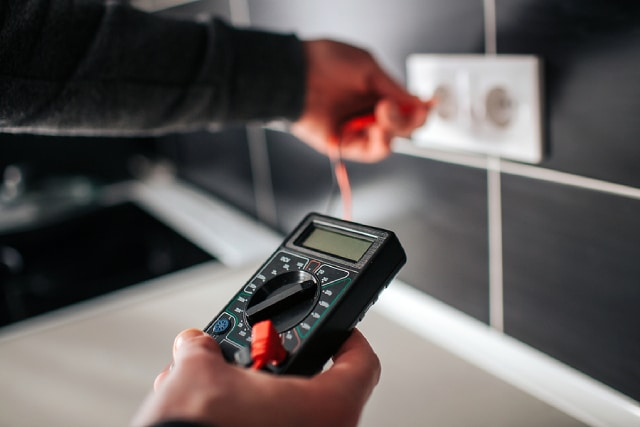The power we use in our residential areas and office buildings typically utilises an alternating current (AC) due to its efficiency. However, there may be instances where a device requires the use of a direct current (DC). In such scenarios, it is possible to convert an alternating current into a direct current.

A rectifier is a device used for converting AC to DC. One or more diodes are installed in this device to allow current to flow in one direction but not the other. Let us explore three examples, and the diodes’ function in converting AC input voltage to DC output voltage.

Half-wave rectifier

A half-wave rectifier is a basic rectifier that utilises only one diode. The device permits only one half-cycle of an AC input voltage to flow through and be converted to DC output voltage, as seen in the diagram below.

Diagram of a half-wave rectifierAn AC voltage is directed through the circuit’s primary side before flowing to the secondary portion of the circuit where the diode is situated. During the positive half-cycle, the diode will be forward biased and allow the current to flow through to be converted to DC output voltage.

The diode will be reverse biased during the negative half-cycle of the AC voltage, and this current is subsequently blocked. This example is seen in the diagram above as only the positive voltage appears at the output. While a half-wave rectifier may be simple to set up, it is inefficient as the DC output voltage generated is roughly half of the AC input voltage.

Full-wave rectifier

For a more efficient and steady output, we can instead look to utilise a full-wave rectifier.

Diagram of a full-wave rectifierA full-wave rectifier utilises two diodes that are connected to the outer terminals. There is a centre tap that is used as the neutral ground for the rectified DC voltage. Similar to the half-wave rectifier, an AC input voltage is directed through the primary side of the circuit.

During the positive half-cycle of the voltage, the first terminal is positively charged while the second terminal is negatively charged. As a result, the diode D1 will be forward biased, and the current will flow through and be converted to DC output voltage. Diode D2 is reverse biased and will block the current.

The reverse will be true during the negative half-cycle of the AC voltage. The first terminal is negative, and the second vice-versa. Diode D2 will be forward biased, with the current flowing through the second diode instead. Diode D1 is reverse biased and will block the current. This results in both half-cycles passing through, so the DC output voltage generated is greater than that of a half-wave rectifier.

Bridge rectifier

Despite the increased output from a full-wave rectifier, it is still relatively inefficient due to the centre-tapped transformer’s presence. The full-wave rectifier is only able to produce roughly half of the total output voltage of the transformer.

We can correct this issue with a bridge rectifier that utilises four diodes arranged in a diamond pattern, as seen in the diagram below.

Diagram of a bridge rectifierDuring the positive half-cycle of the AC voltage, the first terminal is positively charged, which causes the diodes D1 and D3 to turn forward biased. The second terminal is negatively charged, and the diodes D2 and D4 will be reverse biased. The blockage caused by D2 and D4 results in the current flowing through the path created by D1 and D3.

The inverse happens during the negative half-cycle, with the second terminal turning positive. Diodes D2 and D4 will be forward biased to allow the current to flow through, with diodes D1 and D3 functioning as blockers now.

Without the presence of a centre-tapped transformer, the DC output voltage is magnified. A bridge rectifier is the most efficient way to convert AC to DC among the three examples shown.

Conclusion

There are many methods available for one to convert AC to DC. It can be fun to experiment and discover the various ways one can generate a DC output voltage. Experimentation is essential to learning how the universe works, and that is what physics is all about.

If you are interested to learn more but find yourself struggling with the subject, you may want to consider physics tuition. We offer both O level physics tuition and H2 Physics tuition classes. Sign up for our lessons now! Our experienced instructors can show you the fundamentals and ensure you ace the subject